# Examples for secondary school students - page 54

1. Threesome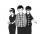Dana, Dalibor and Michael have a combined 57 years. Dana is five years older than Dalibor, but Dana is five years younger than Michael. Determine how old is Dana, Dalibor and Michael.
2. Four integersFnd four consecutive integers so that the product of the first two is 70 times smaller than the product of the next two.
3. Internal anglesFind the internal angles of the triangle ABC if the angle at the vertex C is twice the angle at the B and the angle at the vertex B is 4 degrees smaller than the angle at the vertex A.
4. Repair company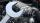The company repairs cars. The first day repair half of the contract second day, the half of the rest and third day 8 residue cars. How many total cars company repaired?
5. Tenth memberCalculate the tenth member of geometric sequence when given: a1=1/2 and q=2
6. The swing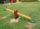To swing the two girls. Aneta weight 45 kg and Simon 35 kg weight. How far should sit Simon from the middle of swing so it is balanced, if we know that Aneta is sitting at distance 1,5m? How far are girls sitting apart?
7. Probability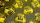What are the chances that the lottery, in which the numbers are drawn 5 of 50 you win the first prize?
8. VariableFind variable P: PP plus P x P plus P = 160
9. Daily temperature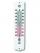The average of daily temperature measurements in one week every day at the same hour was -2.8 °C. All temperatures were measured in different days are different. The highest daily maximum temperature was 2.4 °C, the lowest -6 °C. Determine the options tha
10. Candy - MOGretel deploys to the vertex of a regular octagon different numbers from one to eight candy. Peter can then choose which three piles of candy give Gretel others retain. The only requirement is that the three piles lie at the vertices of an isosceles triang
11. Electricity consumption cost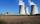Last year Karol family reduced its electricity consumption by 31% compared to the previous year and paid CZK 2883 less. How many CZK is electricity last year and how many two year ago?
12. Difference of two numberThe difference of two numbers is 20. They are positive integers greater than zero. The first number raised to one-half equals the second number. Determine the two numbers.
13. AP - simpleFind the first ten members of the sequence if a11 = 132, d = 3.
14. Sawmill factory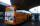Peter works in the factory. The bus stop is 10 km from the factory. Therefore, always when the bus arrives for Peter, the driver leaves factory and takes him to work. They are coming at the saw exactly at 8:00. Today the bus arrived 11 minutes earlier and.
15. Cylinder horizontallyThe cylinder with a diameter of 3 m and a height/length of 15 m is laid horizontally. Water is poured into it, reaching a height of 60 cm below the axis of the cylinder. How many hectoliters of water is in the cylinder?
16. EQ2Solve quadratic equation: ?
17. Inscribed sphereHow many % of the volume of the cube whose edge is 6 meters long is a volume of a sphere inscribed in that cube?
18. Ball gameRichard, Denis and Denise together scored 932 goals. Denis scored 4 goals over Denise but Denis scored 24 goals less than Richard. Determine the number of goals for each player.
19. Permutations without repetitionFrom how many elements we can create 720 permutations without repetition?
20. TreesA certain species of tree grows an average of 0.5 cm per week. Write an equation for the sequence that represents the weekly height of this tree in centimeters if the measurements begin when the tree is 200 centimeters tall.

Do you have an interesting mathematical example that you can't solve it? Enter it, and we can try to solve it.

To this e-mail address, we will reply solution; solved examples are also published here. Please enter e-mail correctly and check whether you don't have a full mailbox.